Mobile QR Code1. (Electrical)

Diffusive memristor, threshold switching, leaky integration-and-fire (LIF), parasitic capacitor, relaxation, conducting filament

## I. INTRODUCTION

The implementation of brain-inspired neural networks with the conventional von Neumann computing architecture incurs a considerable amount of cost to transfer data between the memory and the processor [1,2]. Among such brain-inspired artificial neural networks, spiking neural networks (SNNs) have been demonstrated to have an advantage in terms of energy efficiency because the neuronal units in an SNN are event-driven, i.e., only active when they receive or emit spikes . Furthermore, it was confirmed that the implementation of an SNN circuit using a memristor is more efficient than conventional CMOS in terms of energy consumption, integration density, and simplification of circuits [4-6]. In this case, the memristor is an electrical component and defined as a direct relation between the magnetic flux and charge . Based on this concept, the resistance of the memristor can be changed when voltage is applied to the device.

Memristors can be categorized into two types based on the difference in retention time: nonvolatile and volatile memristors. For nonvolatile memristors, the resistance state can be maintained even without an external voltage; thus, it is a promising candidate for synaptic devices in neuromorphic computing and nonvolatile memory applications. Unlike the nonvolatile memristor, the volatile memristor returns to its initial resistance state when the external voltage is removed. Because of this volatile property, it can be used as a selector for crossbar arrays, artificial neurons in neuromorphic computing, and security applications [5, 8-10].

Recently, volatile memristors have been used to implement neuron nodes, such as integrate-and-fire (IF), leaky IF (LIF), and Hodgekin-Huxley model, to simplify circuits [11-13]. Volatile memristors have been studied by using various materials and mechanisms, such as metal-to-insulator transition, ovonic threshold switch, and diffusive memristors . Among these materials and mechanisms, diffusive memristors have attracted attention owing to their high nonlinearity and unique temporal conductance evolutionary dynamics. These devices are mainly operated by the electromigration/out-diffusion of active metal ions. Active metals, such as Cu and Ag, are present in devices made of metal-dielectric-metal stacks, where active metals can be used as metal electrodes and/or embedded in dielectric layers. When a voltage higher than the threshold voltage is applied to the device, threshold switching occurs with the formation of conductive filaments (CFs) made of active metal ions in the dielectric layer. When the applied voltage falls below the hold voltage, the active metal atoms in the CFs diffuse out spontaneously to minimize their surface energy. Such a self-reset process without an additional reset circuit can be highly advantageous for implementing an LIF neuron circuit .

Recently, we developed an electroforming (EF)-free diffusive memristor by controlling the doping concentration of Ag in an Ag-doped HfO$_{\mathrm{x}}$ layer by co-sputtering . Our observation is that the EF-free device with high Ag concentration showed better threshold switching performance than that of the EF-needed device in terms of nonlinearity and off current. EF-free diffusive memristor could be also beneficial compared to the device with active metal electrode, such as Ag/HfO$_{2}$/Pt, where either EF process or additional activation process is required [16-18]. In this study, a diffusive memristor based on a Pt/Ag-doped HfO$_{\mathrm{x}}$/Pt device was fabricated, and its volatile switching dynamics were investigated. The effects of parasitic elements were observed by performing pulse measurements. Based on the understanding on charging and relaxation characteristics, the LIF neuronal function was demonstrated by using the device and series resistors. A more simplified model of an LIF circuit with this diffusive memristor is proposed in this study.

## II. METHODS

28-nm-thick Ag-doped HfO$_{\mathrm{x}}$ switching layer was deposited on a Pt/Ti/SiO$_{2}$/Si substrate by the co- sputtering of Ag and HfO$_{2}$ targets for 5 min with a sputtering power of 12 W for Ag and 150 W for HfO$_{2}$.

##### Fig. 1. Schematics of the (a) co-sputtering system of Ag-doped HfO$_{2}$ layer; (b) Threshold switch device under test (DUT); (c) pulse measurement system.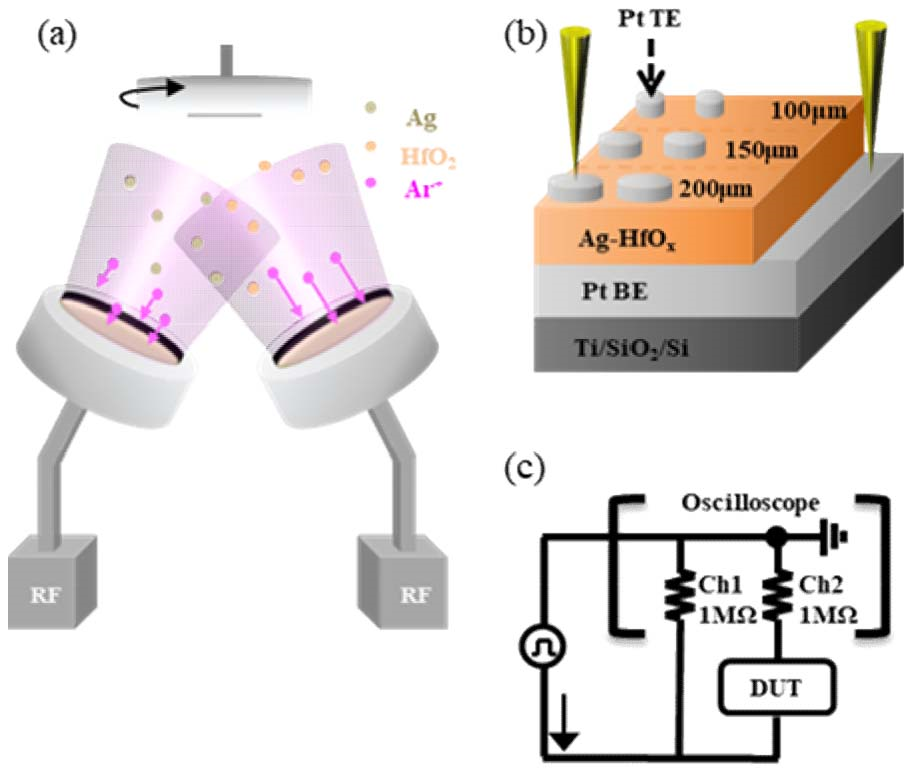Fig. 1(a) shows the schematics of the co-sputtering system. The base pressure was set to ~5x10$^{-6}$ Torr, and the process pressure was 10 mTorr. To maintain the Ar plasma, 20 sccm of Ar gas was let into the chamber. Subsequently, a Pt top electrode was deposited on an as-deposited Ag-doped HfO$_{\mathrm{x}}$ switching layer by employing e-beam evaporation using shadow masks having diameters of 100, 150, and 200~${\mu}$m. A schematic of the device under test (DUT) of the Pt/Ag-doped HfO$_{\mathrm{x}}$/Pt stack is shown in Fig. 1(b). Electrical tests were performed by using a semiconductor parameter analyzer (SPA; HP-4155A), an oscilloscope (OSC; TDS-3032B), and a function generator (FG; AFG-3102) at about 25 ℃. Quasi-DC current-voltage (I-V) threshold switching curves were measured by using the SPA. Waveforms of the voltage pulse and current passing through the DUT were measured by using the equivalent circuit shown in Fig. 1(c); the input voltage waveform was measured in Ch1 of the OSC and the output current waveform was measured in Ch2. The impedance of Ch1 and Ch2 was set to 1 M${\Omega}$ to ensure correct measurement of the applied voltage and current across the internal resistor of the OSC.

Auger electron spectroscopy (AES; XE-100) and X-ray photoelectron spectroscopy (XPS; PHI 5000 with Al K$_{\mathrm{\alpha }}$) were used for the chemical analysis of the switching layer.

## III. RESULTS

The chemical bonding state and depth elemental profiles of the Ag-HfO$_{\mathrm{x}}$ layer were analyzed. Fig. 2(a) and (b) show the XPS spectra of Hf 4f and O 1s from the as-grown HfO$_{2}$ and Ag-doped HfO$_{\mathrm{x}}$ layers. It was confirmed that the binding energy of HfO$_{2}$ was mainly observed. However, there was a shift (~0.5 eV) in the higher binding energy owing to Ag doping. Fig. 2(c) shows the Ag 3d spectrum, where only metallic Ag bonding is observed in the Ag-doped HfO$_{\mathrm{x}}$ layer. Fig. 2(d) shows the AES depth elemental profiles of the switching layer. According to the results, Ag appears uniformly inside the film. However, there is slightly more Ag distributed near the surface and bottom interface .

##### Fig. 2. XPS spectra of (a) Hf 4f; (b) O 1s in HfO$_{2}$ (upper panel) and Ag-doped HfO$_{\mathrm{x}}$ layer (lower panel); (c) Ag 3d spectrum in Ag-doped switching layer; (d) AES depth elemental profiles in Ag-doped HfO$_{\mathrm{x}}$ layer on Si substrate.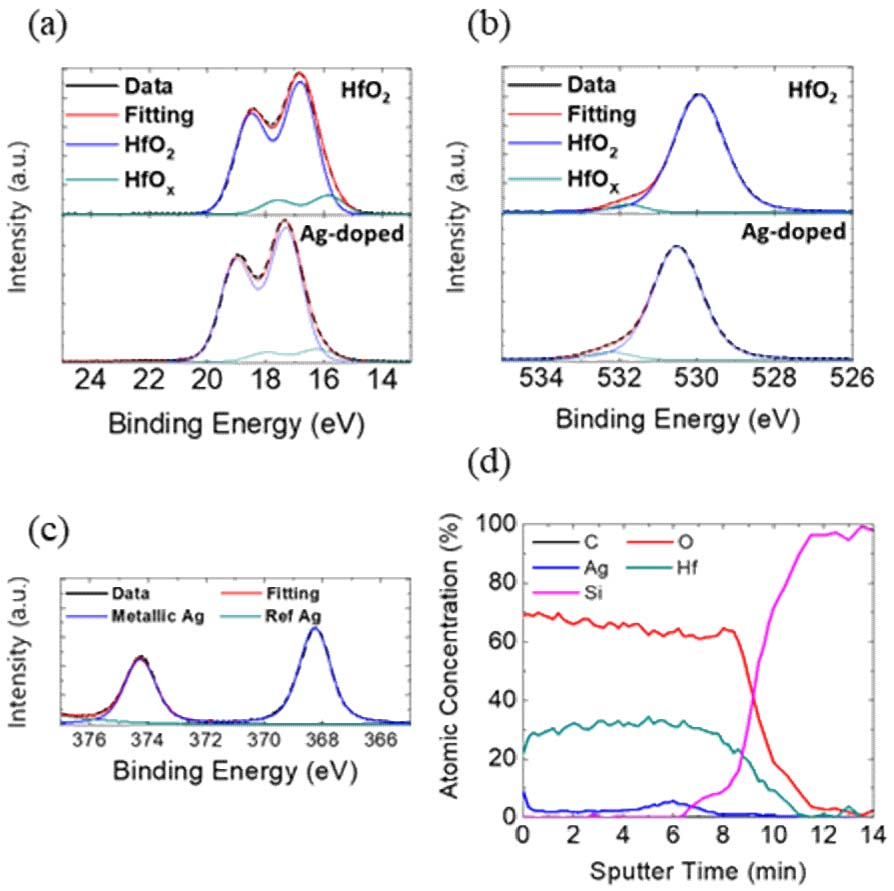##### Fig. 3. (a) Typical DC I-V curves; (b) An example of voltage and current waveforms, where rising, width, and falling times of the input pulse are set to 400 ${\mu}$s, 2 ms, and 400 ${\mu}$s, respectively. Normalized voltage and current waveforms are shown for 1-10 V of input voltages with pulse widths of (c) 2.4~ms; (d) 240 ${\mu}$s.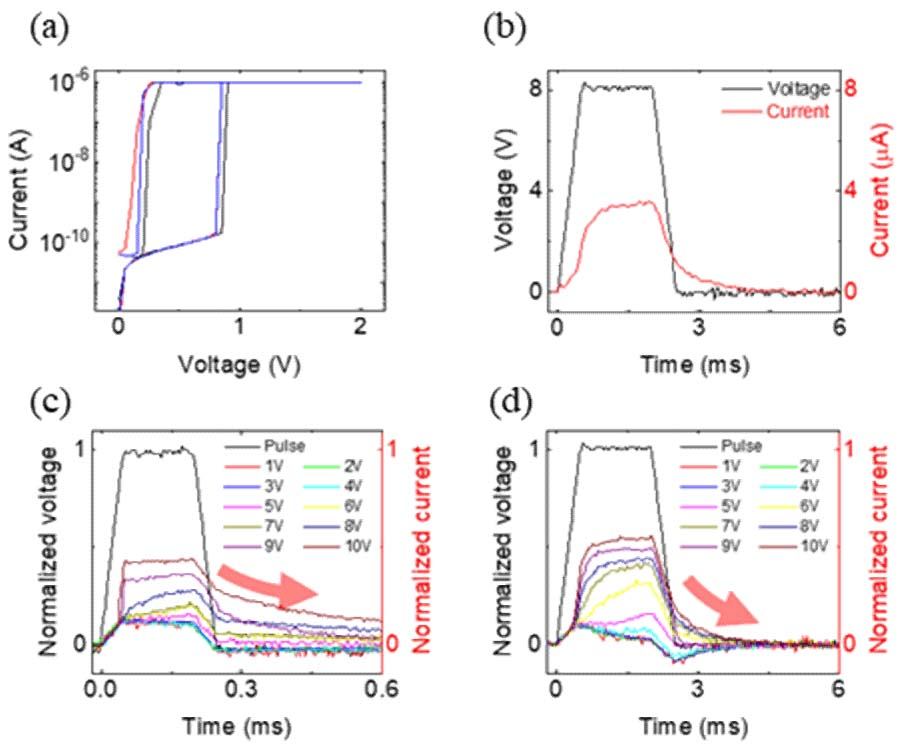The electrical properties of the Pt/Ag-doped HfO$_{\mathrm{x}}$/Pt threshold switch device were analyzed by applying a DC double sweep (sweep rate of approximately 1-10 V/s) and short voltage pulse. The typical I-V curves of the threshold switch are presented in Fig. 3(a). Volatile threshold switching characteristics were observed, as previously reported . The threshold voltage (V$_{\mathrm{th}}$) was approximately 0.8 V, and a large on/off ratio (>10$^{4}$) was observed. Here, we set the current compliance to 1 ${\mathrm{\mu}}$A to prevent excessive current flow. It was also a nonpolar switching behavior, indicating that threshold switching could occur by both bias polarities. Moreover, in this study, pulse measurements were performed. To confirm the charging/discharging effect of threshold switching during the pulse rising and falling, the pulse time ratio of rising, width, and falling was set to 1:5:1. Threshold switching was observed by comparing the input voltage (black line) and output current (red line) waveforms, as shown in Fig. 3(b). Initially, there was a time delay between the current surge and rising voltage pulse, where threshold switching occurred over the threshold voltage. When an input voltage pulse of 8 V was maintained, the output current increased slightly, but the threshold switching was maintained. When the voltage pulse decreased in the falling period, the output current slowly decreased, and this is known as the relaxation behavior due to the out-diffusion of active Ag$^{+}$ ions .

To examine the threshold switching behavior as a function of amplitude and pulse time, an alternating input voltage pulse was applied from 1-10 V with 2.4 ms and 240 ${\mu}$s of pulse width. Then, the output current was normalized by dividing the current by the applied voltage value, as shown in Fig. 3(c) and (d). At a relatively low voltage, below 5 V, no threshold switching occurred but capacitive charging characteristics were observed, as shown in Fig. 3(c). In contrast, at a relatively high voltage, above 5 V, threshold switching and relaxation were observed. The higher the input voltage, the higher was the current flow during the pulse input and beginning of relaxation. When the input pulse width decreased from 2.4 ms to 240 ${\mu}$s, as depicted in Fig. 3(d), the input voltage for the successful threshold switching increased to more than 7 V. In other words, a higher voltage is required with a shorter voltage pulse for threshold switching owing to the highly nonlinear switching dynamics of ionic motion [19-21].

##### Fig. 4. (a) Pulse waveforms of input voltage and output current when the applied voltage is below the threshold switching; (b) Schematic diagram of the device with capacitor (C) and series resistor (R). A volatile memristor is connected in parallel for threshold switching; (c) Fitted results of C and R with different electrode sizes.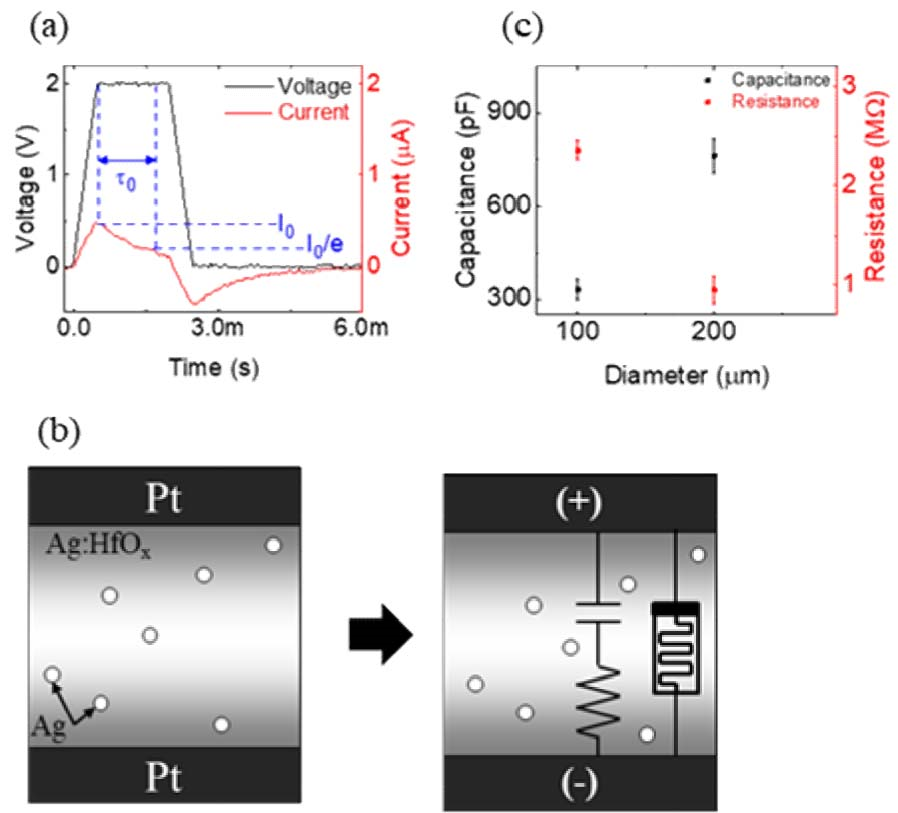To evaluate the capacitive charging characteristics at a relatively low voltage input, as shown in Fig. 4(a), the parasitic capacitance is considered . As shown in Fig. 4(b), capacitive charging before threshold switching is modeled by using an internal capacitor and a series resistor. Here, the capacitor and resistor combination is considered as the device itself with a metal-insulator-metal structure. A volatile memristor is connected in parallel; however, this is not considered before resistance switching. No movement of Ag is expected when a low voltage is applied. This is because the internal parameters were confirmed to remain constant during the pulse application. In the calculation, devices having electrode diameters of 100 and 200 ${\mu}$m were considered. First, to calculate the capacitance (C), the differential equation for the voltage during the pulse rising period (0 < t < t$_{\mathrm{rising}}$) was used as follows.

##### (1)
$V_{S}\left(t\right)=\left(R+1\right)I\left(t\right)+V_{C}\left(t\right)$

V$_{S}$ is the source voltage, V$_{C}$ is the voltage applied to the capacitor, and R+1 is the sum of the resistor and internal resistor impedances of Ch2 (in M${\Omega}$). Based on the definition of capacitance, V$_{S}$ can be defined as follows.

##### (1)
$V_{S}\left(\mathrm{t}\right)=At=\left(R+1\right)C\frac{\mathrm{d}V_{C}}{\mathrm{d}t}+V_{C}$

Here, A is the velocity of the rising pulse. Eq. (2) is solved to derive the following equation:

##### (2)
$I\left(t\right)=AC\left[1-\mathrm{e}^{-\frac{t}{\tau _{0}}}\right],$

##### Fig. 5. (a) Example of relaxation time extraction from the output current waveform; (b) Relationship between I$_{\mathrm{r0}}$ and relaxation time.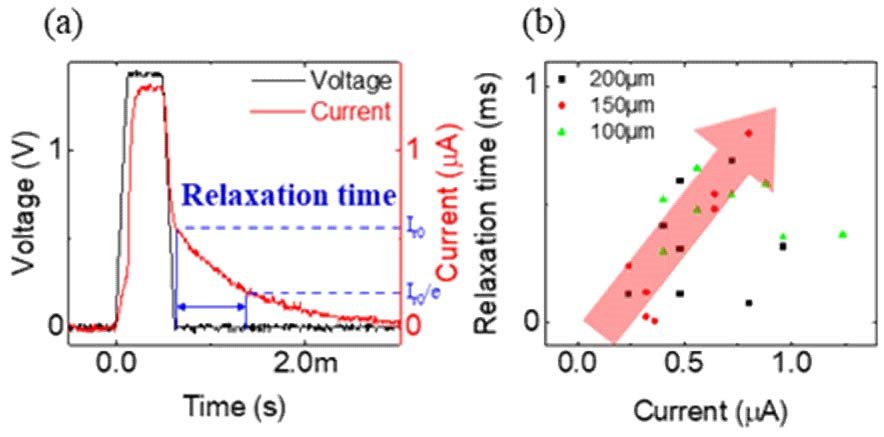##### Fig. 6. (a) Continuous pulse trains of 0.5 (top panel), 0.8 (middle panel), and 1 V (low panel) and their output currents; (b) Waveforms showing the integration and fire of diffusive memristor in (a) 0.8 V; (c) Equivalent LIF circuit containing the diffusive memristor developed in this study.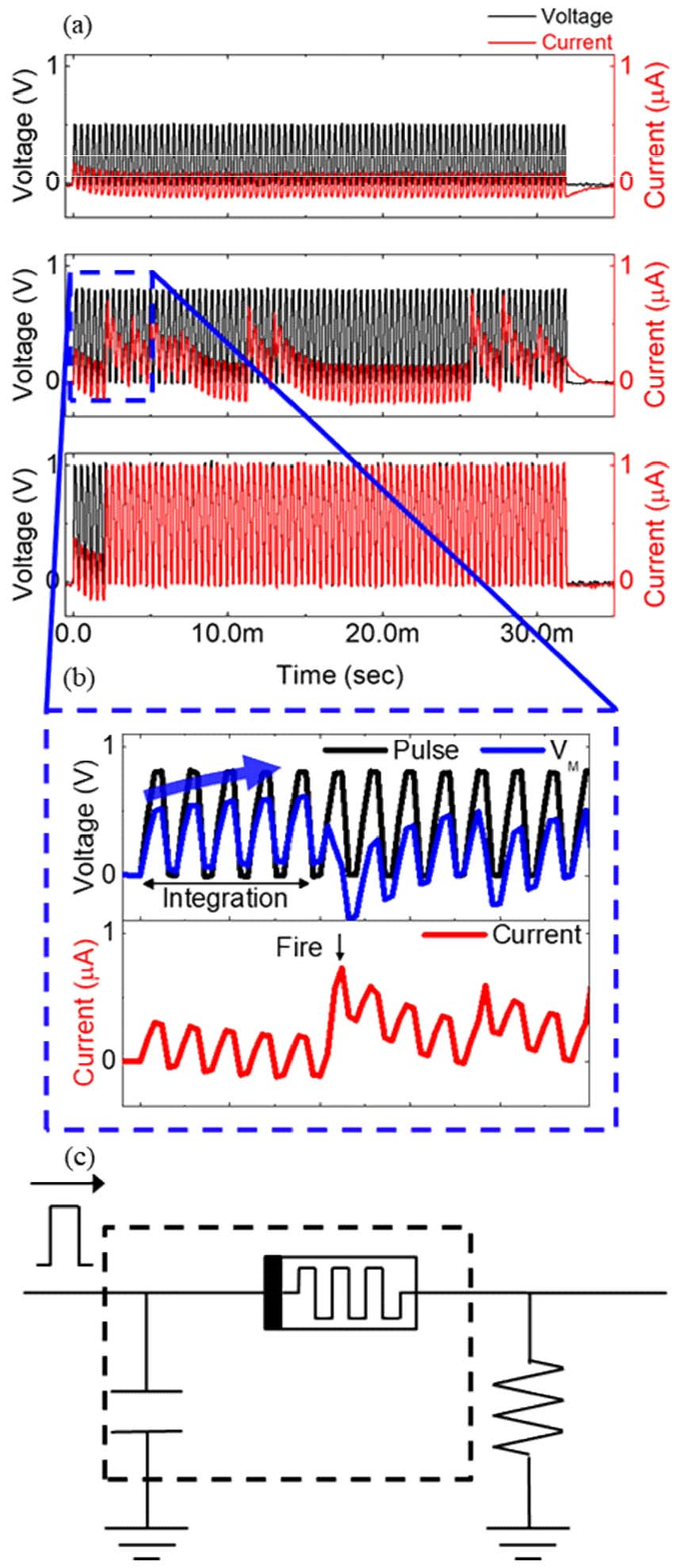Fig. 5(a) shows the relaxation behavior after the threshold switching. Because relaxation is related to the out-diffusion of active ions, the relationship between the initial current of relaxation (I$_{\mathrm{r0}}$) and relaxation time was determined from the waveforms. The current decreased exponentially during relaxation, and the relaxation time was set as the time when I$_{\mathrm{r0}}$ fell below I$_{\mathrm{r0/\mathrm{e}}}$. Fig. 5(b) shows that I$_{\mathrm{r0}}$ is linearly proportional to the relaxation time. There is no dependence on the electrode size; therefore, it is considered that the parasitic capacitor has no effect on the relaxation time. Furthermore, the higher the amplitude and pulse width, the higher were I$_{\mathrm{r0}}$ and the relaxation time. Therefore, the amplitude and pulse width of the input voltage can control the size of the conduction filament and its relaxation .

To verify the charging, threshold switching, and relaxation effects of the diffusive memristor, a continuous voltage pulse train was applied to the device, as shown in Fig. 6(a). The amplitude of the input pulse was varied between 0.5, 0.8, and 1 V, which is lower than the threshold voltage of the pulse measurement. The pulse width was set to 160 ${\mu}$s along the same interval, and 80 pulses were consecutively applied.

In the introduction of 0.5 V pulse trains, no threshold switching was observed, but capacitive charging characteristics appeared, as presented in the top panel of Fig. 6(a). This is because the applied voltage was not sufficient for the threshold switching. It was also confirmed that the current saturated when the pulse was continuously applied. This indicates that charge (integration) occurs during pulse application, and discharge (leak) occurs during the interval.

Furthermore, integration, threshold switching, and subsequent relaxation were observed by introducing 0.8~V pulse trains in the middle panel of Fig. 6(a). Specifically, Fig. 6(b) shows the voltage applied to the memristor (V$_{\mathrm{M}}$) and output current separately under this condition. Before threshold switching, V$_{\mathrm{M}}$ continuously increased with an increasing number of pulses. In other words, when the pulse train is applied, the memristor is charged (integration) before switching because of parasitic capacitance in the device. This means that the voltage is integrated by the internal capacitance of the device.

Once the voltage charged to the device exceeds the threshold voltage (V$_{\mathrm{M}}$>V$_{\mathrm{th}}$), threshold switching of the device in HRS to LRS (firing) occurs and the rise of current can be found. After switching, relaxation process is observed due to the out-diffusion of Ag ions. Pulse trains are applied during the relaxation, but threshold switching does not occur immediately. This is because V$_{\mathrm{M}}$ was not yet sufficiently applied owing to the relaxation effect.

During the relaxation, the device still has a lower resistance compared to R$_{\mathrm{ext}}$, so that V$_{\mathrm{M}}$ is not enough to switch back to LRS over V$_{\mathrm{th}}$. Once the relaxation process is completed and the resistance of the device recovered to HRS, the integration and firing process occurred again by applying the pulse trains.

When 1 V pulse trains were introduced, however, threshold switching was observed but was not followed by relaxation as shown in the bottom panel of Fig. 6(a). In other words, this device did not return to its high-resistance state. The energy of the CF was stabilized and maintained in a low-resistance state .

Fig. 6(c) shows the simplified LIF neuron circuit with a diffusive memristor. It was confirmed that the diffusive memristor could act as a capacitor and memristor. The integration process is related to the charging of the internal capacitor, and threshold switching above a certain voltage can induce the firing process. It is considered that the timing of the CF formation and its relaxation is comparable to the charging/discharging of the internal capacitor. Therefore, it is important to match the input pulse condition to control the memristor-enabled LIF circuit more precisely.

Lastly, it is concerned that reliability of diffusive memristor can be problematic in terms of retention and endurance. The devices showed reproducible switching characteristics up to more than a year. Whereas DC endurance should be further improved. Scalability of our diffusive memristor was confirmed down to the device with contact-hole diameter of 120 nm. Both reliability and scalability should be important to realize the LIF neuron application.

## IV. CONCLUSION

In this study, a diffusive memristor with a Pt/Ag-doped HfO$_{\mathrm{x}}$ stack was fabricated. Volatile threshold switching was analyzed by performing DC and pulse measurements. A parasitic capacitor and resistor were considered to evaluate the charging characteristics of the diffusive memristor before its threshold switching. The relaxation behavior after threshold switching was related to the out-diffusion of the CF (Ag$^{+}$ ions), which was influenced by the pulse amplitude and width.

Based on the understanding on the charging and relaxation of diffusive memristors, integration and firing processes were observed by applying continuous pulse trains. Under proper pulse conditions, an LIF neuronal function can be implemented by using a single diffusive memristor. Its scalability and controllability are the remaining issues, but it is highly desirable to simplify the circuit and reduce the energy need of an LIF neuron.

## ACKNOWLEDGMENTS

This work was supported by the National Research Foundation of Korea (NRF) grant funded by the Korea government (MSIT) (NRF-2020R1F1A1076232 & 2020M3F3A2A01082324). This work was also supported by the research grant of the Office of Naval Research Global under Grant N62909-20-1-2021-P00001 (Memristor-based Neural Network Circuits).

## References

1
Gao L., Chen P. Y., Yu S., Sep. 2017, NbOx based oscillation neuron for neuromorphic computing, Appl. Phys. Lett., Vol. 111, No. 10, pp. 103503-undefined2
Zidan M. A., Strachan J. P., Lu W. D., Jan. 2018, The future of electronics based on memristive systems, Nat. Electron., Vol. 1, No. 1, pp. 22-293
Roy K., Jaiswal A., Panda P., Nov. 2019, Towards spike-based machine intelligence with neuromorphic computing, Nat., Vol. 575, No. 7784, pp. 607-6174
Zhang Y., et al. , Dec. 2018, Highly Compact Artificial Memristive Neuron with Low Energy Consumption, Small, Vol. 14, No. 515
Wang R., et al. , Sep. 2020, Recent Advances of Volatile Memristors: Devices, Mechanisms, Applications, Advanced Intelligent Systems, Vol. 2, No. 9, pp. 20000556
Wang Z., et al. , Jan. 2017, Memristors with diffusive dynamics as synaptic emulators for neuromorphic computing, Nature Materials, Vol. 16, No. 1, pp. 101-1087
Chua L. O., 1971, Memristor-The Missing Circuit Element, EEE TRANSACTIONS ON CIRCUIT THEORY, Vol. 18, No. 5, pp. 5078
Zhang X., et al. , Dec. 2020, An artificial spiking afferent nerve based on Mott memristors for neuro-robotics, Nat. Comm., Vol. 11, No. 1, pp. 51-undefined9
Xia Q., Yang J. J., Apr. 01. 2019, Memristive crossbar arrays for brain-inspired computing, Nat. Mater., Vol. 18, No. 4. nature publishing group, pp. 309-32310
Wang Z., et al. , Feb. 2018, Threshold Switching of Ag or Cu in Dielectrics: Materials, Mechanism, Applications, Advanced Functional Materials, Vol. 28, No. 611
Tuma T., Pantazi A., le Gallo M., Sebastian A., Eleftheriou E., Aug. 2016, Stochastic phase-change neurons, Nature Nanotechnology, Vol. 11, No. 8, pp. 693-69912
Zhang X., et al. , Feb. 2018, An Artificial Neuron Based on a Threshold Switching Memristor, IEEE Electron Device Letters, Vol. 39, No. 2, pp. 308-31113
Pickett M. D., Medeiros-Ribeiro G., Williams R. S., 2013, A scalable neuristor built with Mott memristors, Nature Materials, Vol. 12, No. 2, pp. 114-11714
Lee D., et al. , Sep. 2019, Various Threshold Switching Devices for Integrate and Fire Neuron Applications, Advanced Electronic Materials, Vol. 5, No. 915
Jeong W. H., Han J. H., Choi B. J., 2020, Effect of Ag Concentration Dispersed in HfOx Thin Films on Threshold Switching, Nanoscale Research Letters, Vol. 15, No. 116
Chekol S. A., Cüppers F., Waser R., Hoffmann-Eifert S., 2021, An Ag/HfO2/Pt Threshold Switching Device with an Ultra-Low Leakage (< 10 fA), High On/OffRatio (> 1011), and Low Threshold Voltage (< 0.2 V) for Energy-Efficient Neuromorphic Computing, 2021 IEEE International Memory Workshop (IMW), pp. 1-417
Kim J, Nam K, Chung H, 2014, Improved Uniformity of Resistive Switching Characteristics in Ag/HfO 2 /Pt ReRAM Device by Microwave Irradiation Treatment, Journal of the Korean Institute of Electrical and Electronic Material Engineers, Vol. 27, No. 2, pp. 81-8418
Grisafe B., Jerry M., Smith J. A., Datta S., Oct. 2019, Performance Enhancement of Ag/HfO2 Metal Ion Threshold Switch Cross-Point Selectors, in IEEE Electron Device Letters, Vol. 40, No. 10, pp. 1602-160519
Strukov D. B., Williams R. S., Nov. 2008, Exponential ionic drift: fast switching and low volatility of thin-film memristors, Appl. Phys. A: Mater. Sci. Proc., Vol. 94, No. 3, pp. 515-51920
Torrezan A. C., Strachan J. P., Medeiros-Ribeiro G., Williams R. S., Dec. 2011, Sub-nanosecond switching of a tantalum oxide memristor., Nanotechnol., Vol. 22, No. 48, pp. 48520321
Menzel S., Waters M., Marchewka A., Böttger U., Dittmann R., Waser R., Dec. 2011, Origin of the Ultra-nonlinear Switching Kinetics in Oxide-Based Resistive Switches, Adv. Func. Mater., Vol. 21, No. 23, pp. 4487-449222
Itoh M., Chua L. O., Jun. 2016, Parasitic Effects on Memristor Dynamics, International Journal of Bifurcation and Chaos, Vol. 26, No. 623
Shukla N., Krishna Ghosh R., Grisafe B., Datta S., 2017, Fundamental mechanism behind volatile and non-volatile switching in metallic conducting bridge RAM, in IEEE International Electron Devices Meeting, pp. 87-90Ju Hwan Park received the B.S. degree in the Department of Materials Science and Engineering from Seoul National University of Science and Technology (Seoultech), in 2022. He is currently pursuing the Master degree at the Advanced Nano Electronics Laboratory, Department of Materials Science and Engineering, Seoultech. His current research interests include Neuromorphic devices.

Won Hee Jung received the B.S. and M.S. degrees in the Department of Materials Science and Engineering from Seoul National University of Science and Technology (Seoultech) in 2018 and 2020, respectively. In 2020, he joined at Samsung Electronics, where he has been working in the area of product engineering, specialty in etching process development of logic product.

Byung Joon Choi received Ph.D. degree from Seoul National University, Seoul, Korea, in 2009. Since then he worked at the University of Pennsylvania, Philadelphia, PA, as a Postdoctoral Researcher. In 2011, he joined Hewlett-Packard Laboratories, Palo Alto, CA. He has been an Associate Professor at the Department of Materials Science and Engineering, Seoul National University of Science and Technology (Seoultech) since 2013. His research fields are development of thin film processes applying for next-generation memory and Neuromorphic devices.